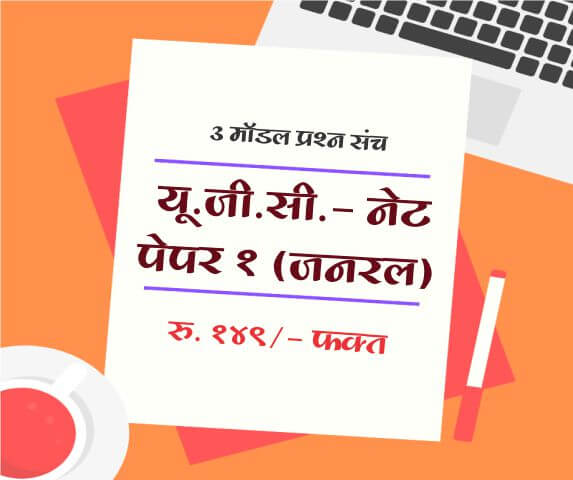# Problems on Percentage

1 What is Percentage?

Percentage is an important topic of quantitative aptitude, Percentage is represented by % symbol and it means values per hundred.

Let’s consider following example

40% means 40 out of 100 and mathematically represented as 40/100.

Simple Percentage Calculator
2 Percentage Increase

Percentage increase is calculated when there is increase in initial or base quantity of commodity.

Let’s consider following example

% Increase Calculator
3 Percentage Decrease

Percentage decrease is calculated when there is decrease in initial or base quantity of commodity.

Let’s consider following example

% Decrease Calculator
4 Percentage Increase in Consumption

If price of commodity is decreased X% then to keep expenditure constant consumption is increased. Increase in Expenditure can be calculated using following formula.

Let’s consider following example

% Increase in Consumption Calculator
 Name : Comment :

Subscribe to get latest updates about Exam Schedules, Government Jobs, General Knowledge & Current Affairs and Many more on Whatsapp!###### Aptitude

Full Length Mock Tests
Timer Based Exams
Instant Result and assesment
Detailed analasys of Result##### UGC NET SET Paper 1 Mock Tests

UGC-NET / SET Paper 1 mock test

3 Mock Tests, 150 Questions with Explanations

Rs: 149 Only/-##### LAW MH-CET 2019 (3 YEAR COURSE)

Mock test for MH CET Law 2019 for three year law programme

3 Mock Tests, 450 Questions with Explanations

Rs: 249 Only/-##### यू. जी. सी. - नेट पेपर १ (जनरल)

यू. जी. सी. - नेट पेपर १ (अनिवार्य) सामान्य प्रश्न हिंदीमें

3 Mock Tests, 150 Questions with Explanations

Rs: 149 Only/-